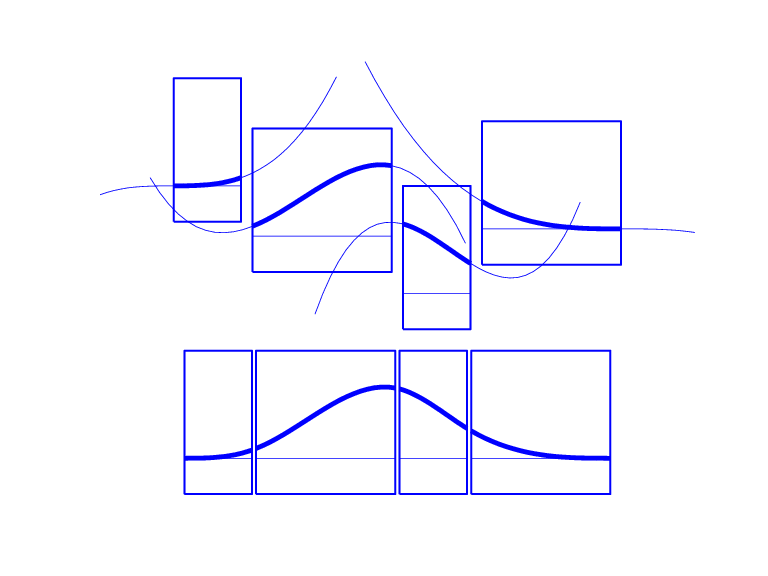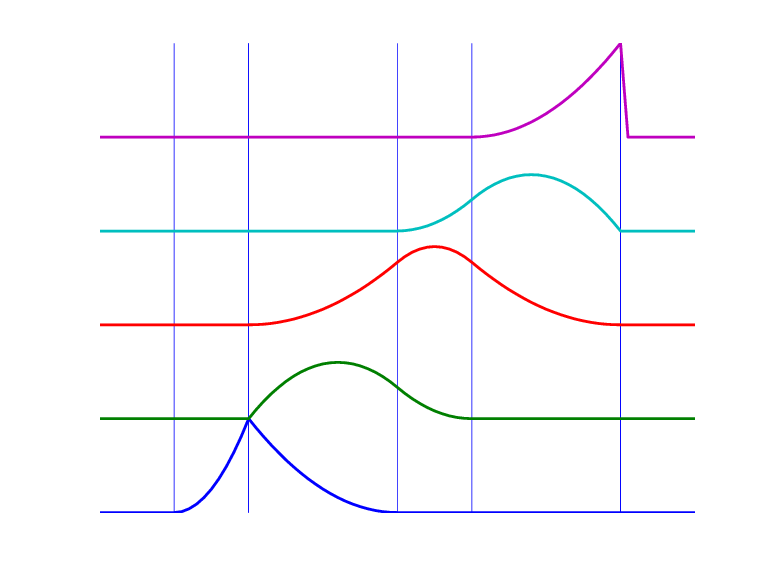## The B-form

### Introduction to B-form

A univariate spline f is specified by its nondecreasing knot sequence `t` and by its B-spline coefficient sequence `a`. See Multivariate Tensor Product Splines for a discussion of multivariate splines. The coefficients may be (column-)vectors, matrices, even ND-arrays. When the coefficients are 2-vectors or 3-vectors, f is a curve in R2 or R3 and the coefficients are called the control points for the curve.

Roughly speaking, such a spline is a piecewise-polynomial of a certain order and with breaks `t`(i). But knots are different from breaks in that they may be repeated, i.e., `t` need not be strictly increasing. The resulting knot multiplicities govern the smoothness of the spline across the knots, as detailed below.

With `[d,n] = size(a)`, and` n+k = length(t)`, the spline is of order `k`. This means that its polynomial pieces have degree <``` k```. For example, a cubic spline is a spline of order 4 because it takes four coefficients to specify a cubic polynomial.

### Definition of B-form

These four items, t, a, n, and k, make up the B-form of the spline f.

This means, explicitly, that

`$f=\sum _{i=1}^{n}{B}_{i,k}a\left(:,i\right)$`

with Bi,k=B(·|t(i:i+k)) the ith B-spline of order `k` for the given knot sequence `t`, i.e., the B-spline with knots t(i),...,t(i+k). The basic interval of this B-form is the interval [t(1)..t(n+k)]. It is the default interval over which a spline in B-form is plotted by the command `fnplt`. Note that a spline in B-form is zero outside its basic interval while, after conversion to ppform via `fn2fm`, this is usually not the case because, outside its basic interval, a piecewise-polynomial is defined by extension of its first or last polynomial piece. In particular, a function in B-form may have jumps in value and/or one of its derivative not only across its interior knots, i.e., across t(i) with t(1)<t(i)<t(n+k), but also across its end knots, t(1) and t(n+k).

### B-form and B-Splines

The building blocks for the B-form of a spline are the B-splines. A B-Spline of Order 4, and the Four Cubic Polynomials from Which It Is Made shows a picture of such a B-spline, the one with the knot sequence `[0 1.5 2.3 4 5]`, hence of order 4, together with the polynomials whose pieces make up the B-spline. The information for that picture could be generated by the command

```bspline([0 1.5 2.3 4 5]) ```

A B-Spline of Order 4, and the Four Cubic Polynomials from Which It Is MadeTo summarize: The B-spline with knots t(i)≤····≤ t(i+k) is positive on the interval (t(i)..t(i+k))and is zero outside that interval. It is piecewise-polynomial of order `k` with breaks at the sites t(i),...,t(i+k). These knots may coincide, and the precise multiplicity governs the smoothness with which the two polynomial pieces join there.

#### Definition of B-Splines

The shorthand

`$f\in {S}_{k,t}$`

is one of several ways to indicate that f is a spline of order `k` with knot sequence `t`, i.e., a linear combination of the B-splines of order `k` for the knot sequence `t`.

A word of caution: The term B-spline has been expropriated by the Computer-Aided Geometric Design (CAGD) community to mean what is called here a spline in B-form, with the unhappy result that, in any discussion between mathematicians/approximation theorists and people in CAGD, one now always has to check in what sense the term is being used.

### B-Spline Knot Multiplicity

The rule is

knot multiplicity + condition multiplicity = order

All Third-Order B-Splines for a Certain Knot Sequence with Various Knot MultiplicitiesFor example, for a B-spline of order 3, a simple knot would mean two smoothness conditions, i.e., continuity of function and first derivative, while a double knot would only leave one smoothness condition, i.e., just continuity, and a triple knot would leave no smoothness condition, i.e., even the function would be discontinuous.

All Third-Order B-Splines for a Certain Knot Sequence with Various Knot Multiplicities shows a picture of all the third-order B-splines for a certain mystery knot sequence `t`. The breaks are indicated by vertical lines. For each break, try to determine its multiplicity in the knot sequence (it is 1,2,1,1,3), as well as its multiplicity as a knot in each of the B-splines. For example, the second break has multiplicity 2 but appears only with multiplicity 1 in the third B-spline and not at all, i.e., with multiplicity 0, in the last two B-splines. Note that only one of the B-splines shown has all its knots simple. It is the only one having three different nontrivial polynomial pieces. Note also that you can tell the knot-sequence multiplicity of a knot by the number of B-splines whose nonzero part begins or ends there. The picture is generated by the following MATLAB® statements, which use the command `spcol` from this toolbox to generate the function values of all these B-splines at a fine net `x`.

```t=[0,1,1,3,4,6,6,6]; x=linspace(-1,7,81); c=spcol(t,3,x);[l,m]=size(c); c=c+ones(l,1)*[0:m-1]; axis([-1 7 0 m]); hold on for tt=t, plot([tt tt],[0 m],'-'), end plot(x,c,'linew',2), hold off, axis off ```

Further illustrated examples are provided by the example ”Construct and Work with the B-form”. You can also use the GUI `bspligui` to study the dependence of a B-spline on its knots experimentally.

### Choice of Knots for B-form

The rule “knot multiplicity + condition multiplicity = order” has the following consequence for the process of choosing a knot sequence for the B-form of a spline approximant. Suppose the spline s is to be of order k, with basic interval [a..b], and with interior breaks ξ2< ·· ·<ξl. Suppose, further, that, at ξi, the spline is to satisfy μi smoothness conditions, i.e.,

`$\begin{array}{ccc}jum{p}_{{\xi }_{i}}{D}^{j}s:={D}^{j}s\left({\xi }_{i+}\right)-{D}^{j}s\left({\xi }_{i-}\right)=0,& 0\le j<{\mu }_{i},& i=2,...,l\end{array}$`

Then, the appropriate knot sequence t should contain the break ξi exactly k – μi times, i=2,...,l. In addition, it should contain the two endpoints, a and b, of the basic interval exactly k times. This last requirement can be relaxed, but has become standard. With this choice, there is exactly one way to write each spline s with the properties described as a weighted sum of the B-splines of order k with knots a segment of the knot sequence t. This is the reason for the B in B-spline: B-splines are, in Schoenberg's terminology, basic splines.

For example, if you want to generate the B-form of a cubic spline on the interval [1 .. 3], with interior breaks 1.5, 1.8, 2.6, and with two continuous derivatives, then the following would be the appropriate knot sequence:

```t = [1, 1, 1, 1, 1.5, 1.8, 2.6, 3, 3, 3, 3]; ```

This is supplied by `augknt([1, 1.5, 1.8, 2.6, 3], 4)`. If you wanted, instead, to allow for a corner at 1.8, i.e., a possible jump in the first derivative there, you would triple the knot 1.8, i.e., use

```t = [1, 1, 1, 1, 1.5, 1.8, 1.8, 1.8, 2.6, 3, 3, 3, 3]; ```

and this is provided by the statement

```t = augknt([1, 1.5, 1.8, 2.6, 3], 4, [1, 3, 1] ); ```